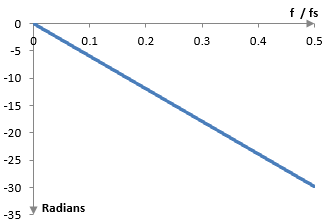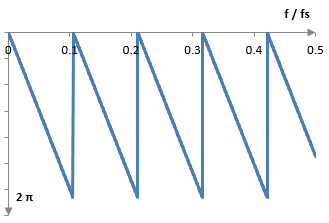# Phase response

The phase response of an equipment or software is a mathematical, numeric, or graphical representation of the difference in phase between the input and output signals for that equipment or software.

The term phase can have several meanings, but for our purposes the phase of a wave is, say, the displacement of the start of the wave's cycle from the start of time. For example, a wave of frequency f can be written as sin(2 π f t), where t is time. The wave starts with a value of zero as at t = 0 we have sin(0) = 0. The wave completes a full cycle between t = 0 and t = 1 / f and at t = 1 / f we have sin(2 π) = 0. Since the cycle of the wave is completed in 1 / f time units, in one time unit the wave will complete f cycles. The wave thus has a frequency of f. This wave's cycle starts at 0. If we want to displace the wave, we can use

$$\sin(2 \pi f (t - T))$$

where T is some constant amount of time. This wave starts its cycle at t = T and hence we can call T the phase of the wave. Alternatively, and more often, we can write the wave as

$$\sin(\omega t – \theta)$$

where ω is the angular frequency &omega = 2 π f and θ is the angular phase θ = 2 π f T. In this case, the displacement of the start of the wave cycle is expressed as an angle (i.e., in radians). For example, since a wave completes a cycle in 2 π, then a phase of θ = π will denote half of the wave's cycle.

Typically, the phase response is the phase shift expressed in radians for different frequencies in the frequency spectrum. Suppose that we send the wave sin(2 π f (t - T1)) through a piece of equipment or software and we get the wave sin(2 π f (t - T2)). We could say that the phase shift is the delay in time between the two waves is T2 – T1. We can also say that, since the wave has a cycle of 1 / f time units, the wave will be delayed by f (T2 – T1) portions of its cycle, or 2 π f (T2 – T1) radians. Similarly, if ω is the angular frequency ω = 2 π f and the phases are θ1 = 2 π f T1 and θ2 = 2 π f T2, then the waves are sin(ω – θ1) and sin(ω – θ2) and the phase response is

$$(\theta_2 – \theta_1) = 2 \pi f (T_2 – T_1)$$

## Why is phase important?

Consider two sine waves with the same frequency f but different phases T1 and T2, say sin(2 π f (t-T1)) and sin(2 π f (t-T2)). We know that

$$\sin(2 \pi f (t-T_1)) + \sin(2 \pi f (t-T_2))$$ $$= 2 \sin(\frac{2 \pi f (t-T_1)+ 2 \pi f (t-T_2)}{2}) \cos(\frac{2 \pi f (t-T_1)- 2 \pi f (t-T_2)}{2})$$ $$= 2 \sin(2 \pi f (t-\frac{T_1+T_2}{2}) \cos(2 \pi f \frac{T_2-T_1}{2})$$

This means that when these two waves are added together the result is a sine wave with the same frequency, but with a phase of (T1+T2) / 2 and with an amplitude that is equal to the amplitude of the original waves scaled by 2 cos(2 π f (T2-T1) / 2). In other words, when two wave with the same frequencies are added together we get a wave with the same frequency, but with amplitude that depends on the two phases and the frequency itself. In the extreme case when the two phases are equal the amplitude will be two times the amplitude of the original waves as 2 cos(0) = 2. In the other extreme case when (T2-T1) = 1 / (2 f) (the two waves have inverted phase as the cycle of the wave is 1 / f time units and 1 / (2 f) is half of the cycle), the waves will cancel each other out as 2 cos(π /2) = 0.

A complex sound wave may consist of many simple waves. If this complex signal is sent to an equipment or software and we get an output signal of a different phase, we should be careful then of adding the input and output signals together. Even if the change in phase (the phase response) is the same across frequencies, it will have a different impact on the amplitude of each frequency and will thus change the frequency content of the signal.

## Example: phase response of a finite impulse response digital low pass filter

It can be shown that a finite impulse response digital low pass filter the coefficients of which are symmetric around the middle delays the input signal by (N – 1) / 2 samples, which is equal to (N – 1) / (2 fs) seconds, where fs is the sampling frequency. This is the filter's phase response. As above, this delay in time is a delay in phase of 2 π f (N – 1) / (2 fs) radians. This makes sense, since as f increases, the wave cycle becomes smaller and the same number of samples is equivalent to a larger delay in angle. The most interesting part of this computation is that the phase shift is linear with respect to f / fs. Thus, this filter is said to have "linear phase".

The phase response of an example filter with N = 20 is shown below. Note that this phase response is shown with respect to (f / fs) on the horizontal axis. According to the Nyquist-Shannon-Kotelnikov sampling theorem, f / fs cannot exceed 0.5. Note also that the phase response is negative, signifying the delay.Since a frequency is just a sine wave however, a delay of, say, 2 π is the same of a delay of zero. A delay of 2 π means that the wave will start exactly a cycle later, which means that it will be equivalent to a wave that is not delayed at all. Since sine waves are periodic, we can remove the full cycles from the phase response delay. For example, a delay of 10 radians is a delay of 10 = 2 π + 3.71, which is equivalent to a delay of 3.71 radians. We can thus also present the phase response as follows.## Phase response given a transfer function

The phase response of a system with transfer function H, at the frequency ω, is

$$\Phi(\omega) = \mathrm{arg}(H)$$

This follows directly from the definition of the transfer function (see Transfer function)). The "arg" function returns the angle between the complex number H and the horizontal axis. For example, when the Re{H} > 0 then arg{H} = arctan( Im{H} / Re{H} ).

## Phase response of a finite impulse response filter with symmetric coefficients

Take a finite impulse response filter with coefficients a(k) that are symmetric around the middle (i.e., a(k) = a(N – 1 – k), k = 0, …, N-1).

This filter computes its output signal y(n) from the input signal x(n) with the formula

$$y(n)= \sum_{k=0}^{N-1} a(k) \, x(n-k)$$

and its Z transform transfer function H(z), evaluated at frequencies with magnitude 1 (at z = ej ω), is

$$H(e^{j \, \omega} )= \sum_{k=0}^{N-1} a(k) \, z^{-k}= \sum_{k=0}^{N-1} a(k) \, e^{-j \,k \, \omega}$$ $$= \sum_{k=0}^{N-1} a(k) \, \cos(k \, \omega) - j \sum_{k=0}^{N-1} a(k) \, \sin(k \, \omega)$$

Then

$$\Phi(\omega) = \mathrm{arctan}(\frac{-\sum_{k=0}^{N-1} s(k) \sin(\omega k)}{\sum_{k=0}^{N-1} a(k) \cos(\omega k)}) = - \mathrm{arctan}(\frac{\sum_{k=0}^{N-1} a(k) \sin(\omega k)}{\sum_{k=0}^{N-1} a(k) \cos(\omega k)})$$

(The arg function does not always return the arctan, so the complications here are a bit simplified.) In the sum in the numerator we can take each two terms with equal coefficients a(k) and a(N – 1 – k) and compute

$$a(k) \sin(\omega k) + a(N – 1 – k) \sin(\omega (N – 1 – k))$$ $$= a(k) (\sin(\omega k) + \sin(\omega (N – 1 – k)))$$ $$= 2 a(k) \sin(\omega \frac{N – 1}{2}) \cos(\omega \frac{N – 1 – 2 k}{2})$$

In the denominator, each two terms with equal coefficients similarly produce

$$a(k) \cos(\omega k) + a(N – 1 – k) \cos(\omega (N – 1 – k))$$ $$= 2 a(k) \cos(\omega \frac{N – 1}{2}) \cos(\omega \frac{N – 1 – 2 k}{2})$$

and the phase response becomes

$$\Phi(\omega)= - \mathrm{arctan}(\frac{2 \sin(\omega \frac{N-1}{2})(1+\sum_{k=0}^{(N-1)/2}(a(k) \cos(\omega \frac{N-1-2k}{2}))))}{2 \cos(\omega \frac{N-1}{2})(1+\sum_{k=0}^{(N-1)/2}(a(k) \cos(\omega \frac{N-1-2k}{2}))))})$$

The "1 + " is there to handle the middle coefficient, assuming that the filter has an odd number of coefficients N. If N is even, the computations will be the same, but without "1 + ". Then

$$\Phi(\omega)= - \mathrm{arctan}(\frac{\sin(\omega \frac{N-1}{2})}{\cos(\omega \frac{N-1}{2})})= -\omega \frac{N-1}{2}$$

This is the same result, which was explained in an ad hoc manner above (ω = 2 π f / fs). Thus, the phase response of a finite impulse response filter with coefficients that are symmetric around the middle is linear with respect to the angular frequency ω.

### Filtered HTML

• Freelinking helps you easily create HTML links. Links take the form of [[indicator:target|Title]]. By default (no indicator): Click to view a local node.